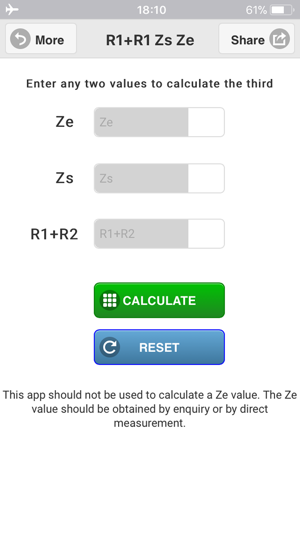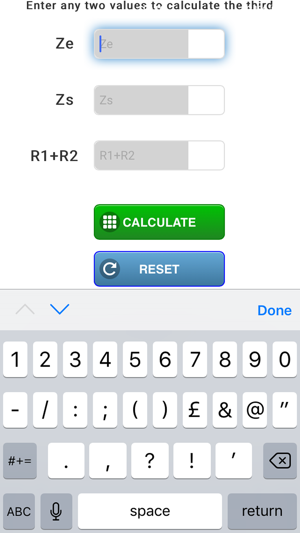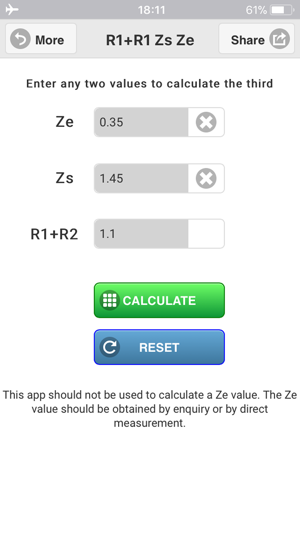## Screenshots

•••## Description

Calculates R1+R2, Zs or Ze. Input any two values to calculate the other.

> Calculate: Zs
> Calculate: (R1+R2)
> Calculate: Ze (not recommended)

Check your measured values with this handy calculator or calculate a value from the other two known values to verify actual measurements obtained.

A fast and effective way to verify and calculate measured values.

Easily calculate the earth loop impedance (Zs) from the R1+R2 and Ze values, also calculate the R1+R2 value from the Zs and Ze values.

A handy app for checking & verifying measured values or calculating a value from the other two known values.

+ Calculate R1+R2
+ Calculate Zs
+ Check you measured R1+R2 readings
+ Validate or verify your Ze

Although this app allows the calculation of the Ze, the Ze should not be calculated, the Ze value should be obtained by actual measurement or by enquiry to the regional electric company (REC).

Remember to check out our full suite of electrical apps such as Cable Calc, Max Zs Values, Ohms Law, PFC Calculator, Watts Amps Volts Calculator, Adiabatic Equation, Electrical Tools & Reference, Earthing & Bonding...

http://www.procertssoftware.com

## What’s New

Version 4.1

Added a share function with QR code. Reduced the App IPA size.

## Ratings and Reviews

2.8 out of 5
14 Ratings

14 Ratings

Astect Gibbo ,

### Not good

I agree with Basic Math if you need this app you definitely need a knew job

Wez261 ,

### Hhmm

As mentioned by "22w" the ze calculation is wrong. This should be measured!!

### Developer Response ,

App now updated for iOS 11 & new UI redesign.

### Basic math

If you need this app to do the calculations this app does, you need to find a new job.
Basic math that most electricians could do in their head.

## Information

Provider
Pro Certs Software Ltd
Size
7.5 MB
Category
Utilities
Compatibility

Requires iOS 8.0 or later. Compatible with iPhone, iPad and iPod touch.

Languages

English

Age Rating
4+
Price
Free

## Supports

•